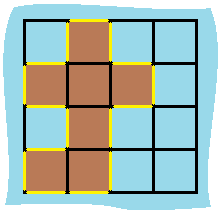# Island Perimeter

Easy
3
34.5% Acceptance

In this lab, you are given a `row x col` `grid` representing a map where `grid[i][j] = 1` represents land and `grid[i][j] = 0` represents water. Your task is to determine the perimeter of the island.

The grid cells are connected horizontally or vertically (not diagonally). The `grid` is completely surrounded by water, and there is exactly one island (i.e., one or more connected land cells).

The island doesn't have "lakes", meaning the water inside isn't connected to the water around the island. One cell is a square with side length 1. The grid is rectangular, width and height don't exceed 100.

## Examples

Example 1:Input: grid = [[0,1,0,0],[1,1,1,0],[0,1,0,0],[1,1,0,0]] Output: 16

In this example, the perimeter is formed by the 16 yellow stripes in the image above.

Example 2:

Input: grid = [] Output: 4

This island consists of a single land cell, and its perimeter is 4.

Example 3:

Input: grid = [[1,0]] Output: 4

In this case, the island consists of a single land cell, and the perimeter is still 4.

## Constraints

• `row == grid.length`
• `col == grid[i].length`
• `1 <= row, col <= 100`
• `grid[i][j]` is `0` or `1`.
• There is exactly one island in `grid`.

## Challenges

1. Export the `islandPerimeter` function. Make sure to export the `islandPerimeter` function using ESM export syntax. The function takes `grid` as an argument and returns the island's perimeter as a number.

2. Calculate the island's perimeter. Implement the `islandPerimeter` function to correctly calculate the island's perimeter based on the given input `grid`.

3. Handle edge cases. Ensure your implementation handles various edge cases like empty grid, grid with a single row or column, and so on.